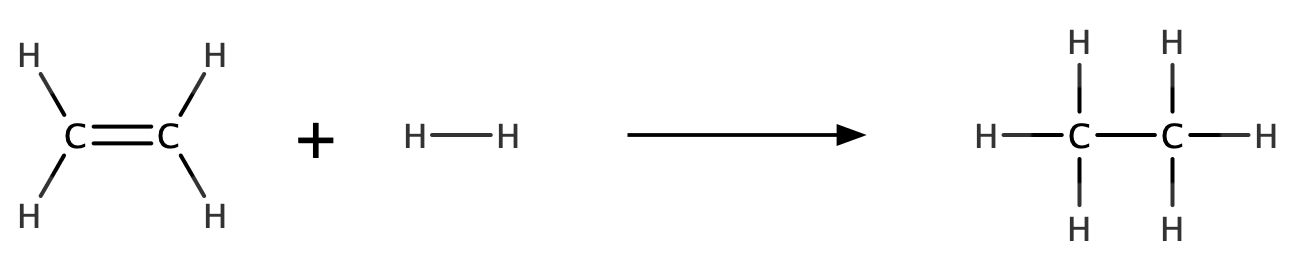# Problem: Hydrogenation reactions are used to add hydrogen across double bonds in hydrocarbons and other organic compounds. Use average bond energies to calculate ΔHrxn for the following hydrogenation reaction: H2C=CH2(g) + H2(g) → H3C–CH3(g)

###### FREE Expert Solution96% (83 ratings)###### Problem Details

Hydrogenation reactions are used to add hydrogen across double bonds in hydrocarbons and other organic compounds. Use average bond energies to calculate ΔHrxn for the following hydrogenation reaction: H2C=CH2(g) + H2(g) → H3C–CH3(g)